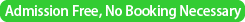### SML Seminar

Date
2nd July 2012 (monday)Time
15:00 -- 18:00
Place
D313, D314 (セミナー室5),
The Institute of Statistical Mathematics
Speaker 1
Matthew Parry (Otago University, New Zealand)
Title
The entropy of scoring rules
Abstract

A scoring rule is a principled way of assessing a probabilistic statement. As such, it finds uses in forecasting and statistical inference. The key requirement of a scoring rule is that it rewards honest statements of ones beliefs.

Associated with each scoring rule is a concave entropy. Conversely, we may (almost) think of each concave entropy as generating a scoring rule. The obvious question is then what features of the entropy are transferred to the scoring rule. I report on recent work on extensive entropies and local entropies. Local entropies are particularly interesting in that they give rise to scoring rules that can assess probability models whose normalization is unknown or is not feasible to compute. I will discuss an application to Bayesian inference for doubly intractable distributions.

Speaker 2
Ben Calderhead (University College London, UK)
Title
A Sample of Differential Geometric MCMC Methods
Abstract

Markov chain Monte Carlo methods enable samples to be drawn from arbitrary probability distributions, and advances in such algorithms have fueled the rapid expansion in the use of Bayesian methodology over the last 20 years. However, one of the enduring challenges in MCMC methodology is the development of proposal mechanisms that make moves distant from the current point, yet are accepted with high probability and at low computational cost.

In this talk I will introduce locally adaptive MCMC methods that exploit the natural underlying Riemannian geometry of many statistical models .Such algorithms automatically adapt to the local correlation structure of the model parameters when simulating paths across the manifold, providing highly efficient convergence and exploration of the target density for many classes of models. I will provide examples of Bayesian inference using these methods on a variety of models including logistic regression, log-Gaussian Cox point processes, stochastic volatility models and Bayesian estimation of dynamical systems described by nonlinear differential equations.

I will then discuss some very recent research in this area, which extends the applicability of Riemannian Manifold MCMC methods to statistical models that do not admit an analytically computable metric tensor.  demonstrate the application of this algorithm for inferring the parameters of a realistic system of highly nonlinear ordinary differential equations using a biologically motivated robust Student-t error model, for which the expected Fisher Information is analytically intractable.

I will conclude with an overview of the outstanding opportunities and challenges that lie ahead at this vibrant intersection between differential geometry and Monte Carlo methodology.

 M. Girolami and B. Calderhead, Riemann Manifold Langevin and Hamiltonian Monte Carlo Methods (with discussion), Journal of the Royal Statistical Society: Series B (Statistical Methodology), 73:123-214, 2011

▲ このページのトップへ